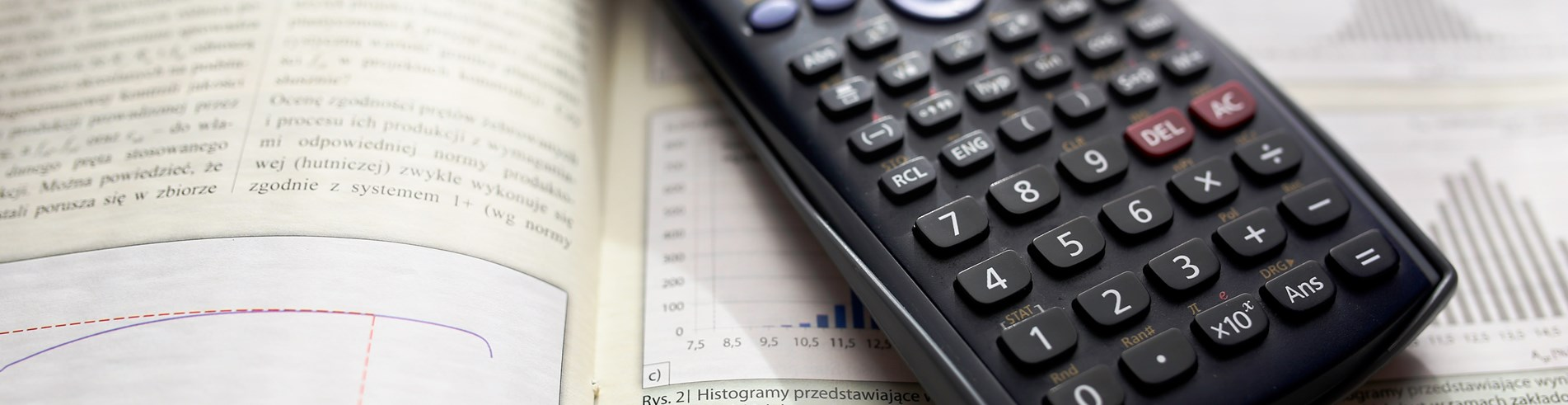Close# Maths

If you look hard enough, you'll see math emerge from some of the most unlikely places. The fact is, we all use math in everyday applications whether we're aware of it or not.

Mathematics is the universal language of our environment, helping mankind explain and create within it for thousands of years. From playing games to playing music, maths is vital to helping students fine tune their creativity and turn their dreams into reality.

#### Potential career paths and further study

Ask any contractor or construction worker - they'll tell you just how important math is when it comes to building anything. To create something of lasting value out of raw materials requires creativity, the right set of tools, and a broad range of mathematics.

Figuring the total amount of concrete needed for a slab; accurately measuring lengths, widths, and angles; and estimating project costs are just a few of the many cases in which math is necessary for real-life home improvement projects.

Some students may say they don't plan on working in construction. While this may be true, many will own a home at some point in their life. Having the ability to do minor home improvements will save a lot of money and headache. Armed with math, these homeowners will also know how to check construction and project estimates, ensuring they're getting the best value.

The opportunity to solve challenging problems is very rewarding. I love discovering new things on my own in maths.
Year 9 student

### MATHS CURRICULUM INFORMATION FOR YEARS 7-11

Year

Term

Units of Work

Assessments

7

Autumn

Number and Calculations

Factors and Multiples

Area and Volume

Charts and Averages

· One topic test per unit.

·Cumulative end of term test.

Spring

Fractions, Decimals and Percentages

Expressions

Shapes and Angles

Ratio

·One topic test per unit.

·Cumulative end of term test.

Summer

Equations

Sequences

Graphs

·One topic test per unit.

·Cumulative end of term test.

8

Autumn

Area and Volume

Expression

Fractions, Decimals and Percentages

Probability

· One topic test per unit.

·Cumulative end of term test.

Spring

Equations

Shapes and Angles

Ratio

Pythagoras and Trigonometry

·One topic test per unit.

·Cumulative end of term test.

Summer

Graphs

Sequences

Charts and Averages

Transformations

·One topic test per unit.

·Cumulative end of term test.

 9 Autumn Factors and Multiples  Number and Calculations Expressions & Equations Charts and Averages · One topic test per unit. ·Cumulative end of term test. Spring Fractions, Decimals and Percentages Ratio Shapes and Angles ·One topic test per unit. ·Cumulative end of term test. Summer Pythagoras and Trigonometry Sequences and Graphs Probability  Transformations ·One topic test per unit. ·Cumulative end of term test. 10 Higher Autumn Cumulative Frequency Equations 2 Area and Volume 2 Graphs ·One topic test per unit. ·Cumulative end of term test. Spring Constructions Equations 3 Circle Theorems · One topic test per unit. ·Cumulative end of term test. Summer Graphs 2 Further Trigonometry Proof and Functions ·One topic test per unit. ·Cumulative end of term test. 10 Foundation Autumn Expressions 2 Area and Volume 2 Graphs Constructions ·One topic test per unit. ·Cumulative end of term test. Spring Fractions, Decimals and Percentages 2 Graphs 2 Equations 2 ·One topic test per unit. ·Cumulative end of term test. Summer Ratio Vectors Equations 3 Transformations · One topic test per unit. ·Cumulative end of term test. 11 Higher Autumn Constructions Equations 3 Circle Theorems ·One topic test per unit. ·Autumn Progress Exam Spring Further Trigonometry Proof and Functions Vectors ·One topic test per unit. ·Spring Progress Exam. 11 Foundation Autumn Equations 2 Ratio 2 Vectors Equations 3 ·One topic test per unit. ·Autumn Progress Exam Spring Pythagoras and Trigonometry Shapes and Angles ·One topic test per unit. ·Spring Progress Exam.

### GCSE COURSE INFORMATION

 Qualification & Board GCSE in Mathematics Foundation – OCR , Higher - Edexcel The assessments will cover the following content headings: 1 Number 2 Algebra 3 Ratio, proportion and rates of change 4 Geometry and measures 5 Probability 6 Statistics Two tiers are available: Foundation and Higher (content is defined for each tier). The qualification consists of three equally-weighted written examination papers One paper is non Calculator and the other two a scientific calculator is permitted Each paper is 1 hour and 30 minutes long. Each paper has a range of question types; some questions will be set in both mathematical and non-mathematical contexts. The qualification will be graded and certificated on a nine-grade scale from 9 to 1 using the total mark across all three papers where 9 is the highest grade. Individual papers are not graded. Foundation tier: grades 1 to 5. Higher tier: grades 4 to 9 (grade 3 allowed). Core 1 1